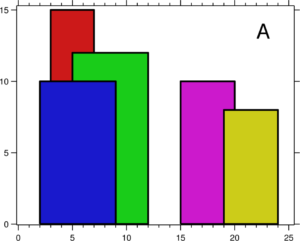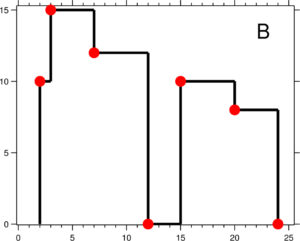# LeetCode_218: The Skyline Problem

Skyline是一个经典问题了，一开始博主思考先进行高度排序，从高到低来确定关键点。事实上通过优先队列来获取当前位置的最大高度，沿着x轴来遍历是最好的。这篇博客提供了一份19ms的答案，战胜了97%，当然是从讨论区淘出来的，博主没想到这么优秀的方法。

### 题目

A city’s skyline is the outer contour of the silhouette formed by all the buildings in that city when viewed from a distance. Now suppose you are given the locations and height of all the buildings as shown on a cityscape photo (Figure A), write a program to output the skyline formed by these buildings collectively (Figure B).The geometric information of each building is represented by a triplet of integers [Li, Ri, Hi], where Li and Ri are the x coordinates of the left and right edge of the ith building, respectively, and Hi is its height. It is guaranteed that 0 ≤ Li, Ri ≤ INT_MAX, 0 < Hi ≤ INT_MAX, and Ri - Li > 0. You may assume all buildings are perfect rectangles grounded on an absolutely flat surface at height 0.

For instance, the dimensions of all buildings in Figure A are recorded as: [ [2 9 10], [3 7 15], [5 12 12], [15 20 10], [19 24 8] ] .

The output is a list of “key points” (red dots in Figure B) in the format of [ [x1,y1], [x2, y2], [x3, y3], ... ] that uniquely defines a skyline. A key point is the left endpoint of a horizontal line segment. Note that the last key point, where the rightmost building ends, is merely used to mark the termination of the skyline, and always has zero height. Also, the ground in between any two adjacent buildings should be considered part of the skyline contour.

For instance, the skyline in Figure B should be represented as:[ [2 10], [3 15], [7 12], [12 0], [15 10], [20 8], [24, 0] ].

Notes:

• The number of buildings in any input list is guaranteed to be in the range [0, 10000].

• The input list is already sorted in ascending order by the left x position Li.

• The output list must be sorted by the x position.

• There must be no consecutive horizontal lines of equal height in the output skyline. For instance, [...[2 3], [4 5], [7 5], [11 5], [12 7]...] is not acceptable; the three lines of height 5 should be merged into one in the final output as such: [...[2 3], [4 5], [12 7], ...]

### 代码

class Solution {
public:
vector<pair<int, int>> getSkyline(vector<vector<int>>& Buildings) {
vector<pair<int, int>> res;
priority_queue<pair<int, int>> active;
int i = 0, n = Buildings.size(), x, y;
while (i < n || !active.empty()) {
x = active.empty() ? Buildings[i] : active.top().second;
if (i >= n || Buildings[i] > x) {
while (!active.empty() && active.top().second <= x) // PQ 中的最大高度的结束点在x之前，全部移除
active.pop();
}
else { // Buildings[i] <= x && i < n // 在处理新的building
x = Buildings[i];
while (i < n && Buildings[i] == x) {  // 对此高度，此起点的所有建筑（可能有多个），都放入 PQ 中
active.push({ Buildings[i], Buildings[i] });
i++;
}
}
y = active.empty() ? 0 : active.top().first;  // 计算关键点的高度
if (res.empty() || res.back().second != y) {  // 刚放进去的元素高度不同
res.push_back({ x, y });
}
}
return res;
}
};